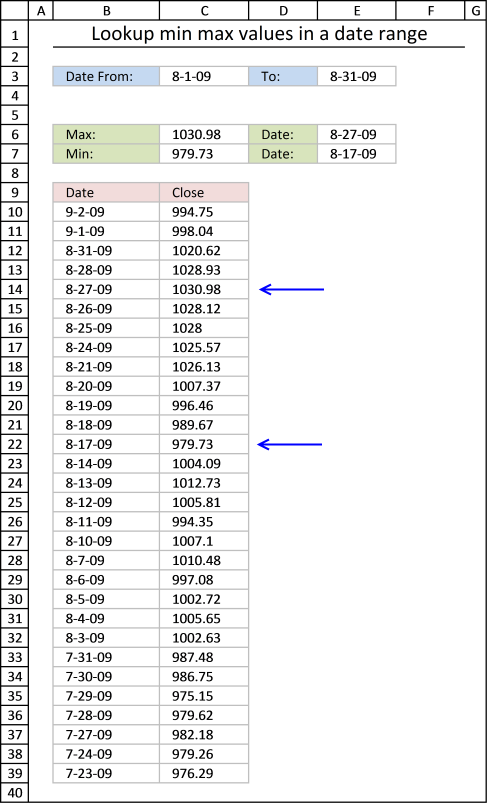Author: Oscar Cronquist Article last updated on December 07, 2018This article explains how to find the smallest and largest value using two conditions. In this case they are date conditions but they can be whatever you like, displayed in cell C3 and E3.

The maximum value in that date range is calculated in cell C6, The minimum value in cell C7. There is also a formula that finds these values and return their corresponding date, in cell E6 and E7.

Formula in cell C6:

=MAXIFS(\$C\$10:\$C\$39, \$B\$10:\$B\$39, "<="&\$E\$3, \$B\$10:\$B\$39, ">="&\$C\$3)

The MAXIFS function returns the largest number from max_range (\$C\$10:\$C\$39) based on a condition or criteria.

MAXIFS(max_rangecriteria_range1criteria1, [criteria_range2criteria2], ...)

The first condition \$B\$10:\$B\$39, "<="&\$E\$3 identifies rows whose date is smaller than or equal to date in cell E3.

The second condition \$B\$10:\$B\$39, ">="&\$C\$3 identifies rows whose date is larger than or equal to date in cell C3.

Formula in cell C7:

=MINIFS(\$C\$10:\$C\$39,\$B\$10:\$B\$39,"<="&\$E\$3,\$B\$10:\$B\$39,">="&\$C\$3)

The MINIFS function works exactly the same as the MAXIFS function, however, the smallest number is instead returned.

Formula in cell E6:

=INDEX(\$B\$10:\$B\$39,MATCH(C6,\$C\$10:\$C\$39,0))

This formula is a simple lookup formula, it returns only a single value from column B if the value in column C matches the contents of cell C6.

INDEX(\$B\$10:\$B\$39,MATCH(C6,\$C\$10:\$C\$39,0))

The MATCH function returns the relative position of a value in a cell range or array.

INDEX(\$B\$10:\$B\$39,MATCH(1030.98,\$C\$10:\$C\$39,0))

becomes

INDEX(\$B\$10:\$B\$39,5)

The INDEX function returns a value based on a row (and column number if needed).

and returns 8-27-09 in cell E6.

Formula in cell E7:

=INDEX(\$B\$10:\$B\$39,MATCH(C7,\$C\$10:\$C\$39,0))